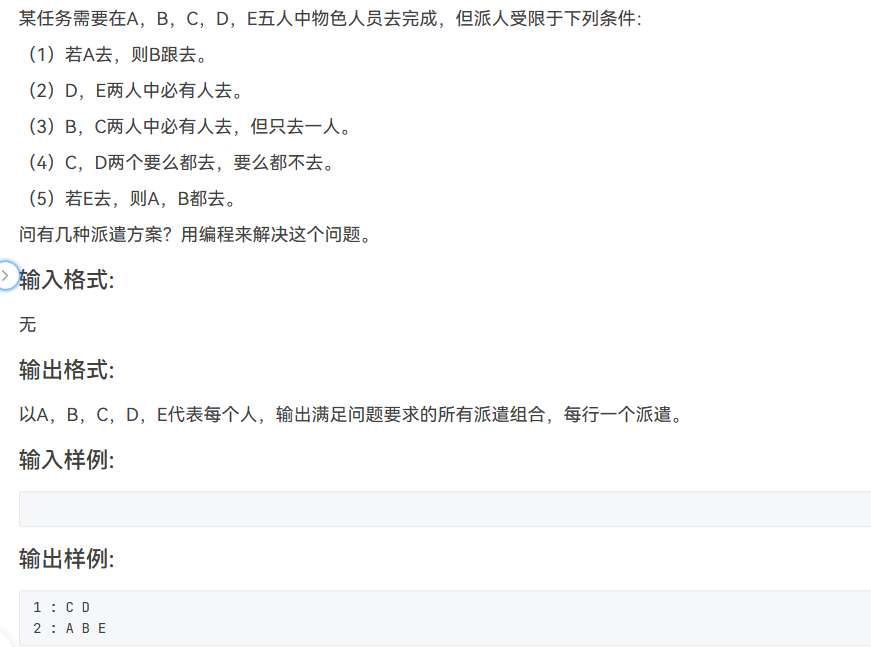# L2-2 人员派遣问题思路讲解：

（1）若A去，则B跟去：

1.A去B也去   A=1,B=1,A+B==2

2.A不去B去   A=0,B=1,A+B==1

3.AB都不去   A=0,B=0,A+B==0

（2）D、E两人中必有人去：

1.DE都去   D=1,E=1,D+E==2

2.D去E不去   D=1,E=0,D+E==1

3.D不去E去   D=0,E=1,D+E==1

（3）B、C两人中必有人去，但只去一人：

1.B去E不去   B=1,E=0,B+C==1

2.B不去E去   B=0,E=1,B+E==1

（4）C、D两个要么都去要么都不去：

1.CD都去   C=1,D=1,C+D==2

2.CD都不去   C=0,D=0,C+D==0

（5）若E去，则A、B都去：

1.E、A、B都去   E=1,A=1,B=1,E+A+B==3

2.E不去，AB随意：E=0

``````#include <stdio.h>
int main()
{
int a,b,c,d,e;
int cnt=0;
for (a=0;a<2;a++){
for (b=0;b<2;b++){
for(c=0;c<2;c++){
for (d=0;d<2;d++){
for (e=0;e<2;e++){
if ( ((a-b)!=1) && ((d+e)!=0) && (b+c==1) && ((c+d==0)|| c+d==2) && ( (e+a+b==3)|| (e==0) ) ){
printf("%d :",++cnt); //输出方案序号
if (a) printf(" A");  //    若a==1则输出A
if (b) printf(" B");
if (c) printf(" C");
if (d) printf(" D");
if (e) printf(" E");
printf("\n");
}
}
}
}
}
}
return 0;
}``````

...全文
39 1 打赏 收藏 转发到动态 举报

1 条回复Scratch3.0趣味编程L2-边玩边学轻松探索美国L1签证申请的常见问题解析2021年安徽省安全员C证考试题库及安徽省安全员C证复审考试Cisco 2800系列集成多业务路由器
v/:* {behavior:url(#default#VML);}o/:* {behavior:url(#default#VML);}w/:* {behavior:url(#default#VML);}.shape {behavior:url(#default#VML);} Normal 0 7.8 磅 0 2优橙国企招聘会—5G网络优化工程师

180发帖与我相关我的任务
c语言c++ 高校 福建省·厦门市• 近7日
• 近30日
• 至今